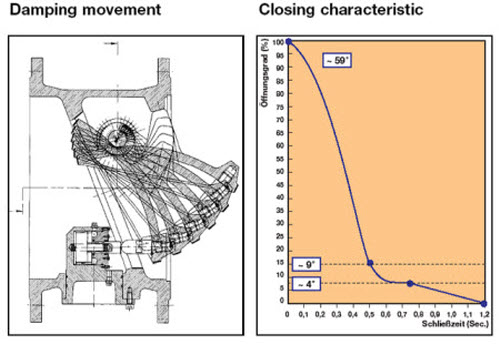# Dynamic Characteristics of Check Valves

Improper sizing of check valves results in check valve slam, a phenomenon that produces undue increase in pressure head due rapid decrease in reverse flow velocity associated with check valve closure. Check valves are placed in pipe systems to prevent flow reversal. However, most check valves require a finite time to activate after sensing flow reversal and the flow could develop significant velocity in the reverse direction before the valve is completely closed. This reverse flow velocity is brought to rest when the valve is closed completely. The rapid change in reverse flow velocity produces a rapid increase in pressure head. The reverse flow velocity at the time of complete closure depends on several factors including the pump inertia, steady state head and flow values in the pipe system, length of liquid column to the source of major wave reflection such as a reservoir, friction losses in piping systems, slope of pipeline, and wave celerity.

Although it is difficult to model the exact closure characteristics of a check valve for lack of reliable manufacturer suggested closure characteristics data, one could verify the validity of check valve modeling assumptions with the help of manufacturer suggested deceleration vs. reverse velocity data.

The proposed check valves for this project are VAG Slanted Seat Swing valves. The manufacturer suggested deceleration vs. reverse velocity data for Slanted Seat Swing check valves is shown in Figure 22. The example calculations shown on this Figure pertain to deceleration calculations following a rapid closure of a control valve at the discharge end of a pipeline. ∆H is the magnitude of pressure wave generated by rapid closure of control valve and L is the pipe length. The deceleration equation was generated by replacing the celerity (c) with L/∆t and rearranging the terms in the Joukowsky’s equation. This example is not applicable for the present modeling study which involves pump failure scenarios and therefore should be ignored. A different approach should be used to calculate deceleration and the associated reverse flow velocities.

Reverse velocity at the time of complete closure of check valve can be assessed based on the deceleration at the time of flow reversal. The deceleration at the time of flow reversal can be determined by analyzing Surge2000 model of the pipeline system with NO check valves at pumps thereby allowing flow reversal. Figure 23 shows the flow velocity at pump discharge following pump trip along with the corresponding deceleration for 2030 flow conditions. From Figure 23, the deceleration at the time of flow reversal is roughly 1.3 m/s2. This deceleration may then be used to determine the potential reverse flow velocity at the time of complete closure of check valve from manufacturer suggested data. Figure 24 shows an approximate reverse flow velocity of 0.05m/s for a deceleration value of 1.3 m/s2. Knowing the celerity (c) of ductile iron pipe (1200m/s) and the velocity change (∆V =0.05m/s), the change in pressure head (pressure spike ∆H) at the time of complete closure of check valve may be determined using Joukowsky’s equation as:

∆H = (c/g) ∆V
∆H = (1200/9.81) 0.05
∆H = 6.11m

This change in pressure head at the time of complete closure may be compared with change in pressure computed by the Surge2000 model to determine if the assumed check valve closure characteristics are reasonable. Figure 25 shows the flow and head variation at pump discharge for the first few seconds of Surge2000 simulation. It may be noted that all Surge2000 models for the pipe system under consideration used a 1 second linear closure time for the check valves. From Figure 25, the change in pressure head at the time of complete check valve closure (zero flowrate though the pump) is around 25m. Since the change in pressure head computed by the Surge2000 model is significantly higher than the value computed from the deceleration characteristics (6.11m), the assumed check valve closure characteristics provide conservative (worse than what’s possible in reality) estimates for the transient pressures. Although deceleration at the time of flow reversal (determined from Figure 23) is an accurate representation for calculating the reversal velocity at the time of complete closure, it may be worthwhile computing reverse velocity based on an average deceleration for further assurance. The average deceleration may be computed based on initial steady state velocity and time for flow reversal as follows.

d = (Vo – 0) / ∆t

This exercise was repeated for current flow conditions. Figure 25a shows the variation of velocity with time. Since the average deceleration results in higher reverse flow velocity, the pressure head change calculations were done with average deceleration only. The average deceleration for current flow conditions is 1.1 m/s2 and the corresponding reverse velocity is 0.04 m/s (Figure 24). The resulting increase in pressure head is 4.9 m (Joukowsky’s Equation) which is also less than the value (25m) predicted by the Surge2000 model.

Figure 26, which shows generic closure characteristics for VAG Slanted Seat Swing Check Valves, also assures that the assumed linear closure characteristics for the check valves will results in conservative estimates for the transient pressures as the actual closure characteristics reduce the flow area very rapidly in the first half of the closure time.

Figure 22

Dynamic characteristics of VAG SKR Slanted Seat (blue lines) non-return valves (courtesy: VAG)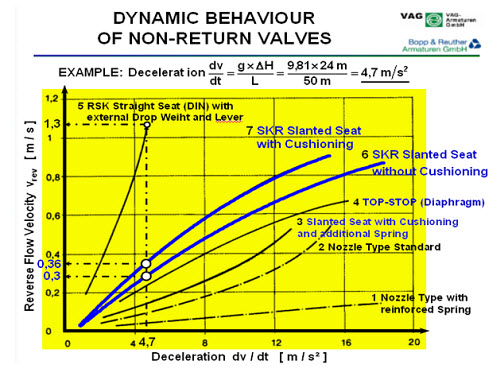Figure 23

Flow deceleration at pump discharge following pump trip – pumps were modeled with NO check valves.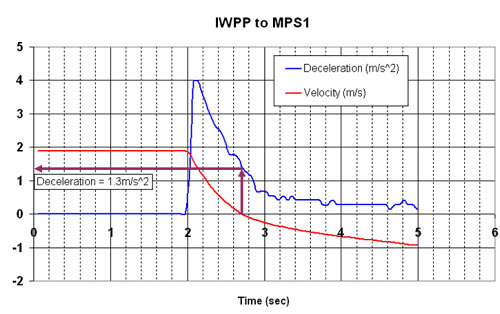Figure 24

Enlarged view of Figure 22 showing expected reverse velocity based on calculated deceleration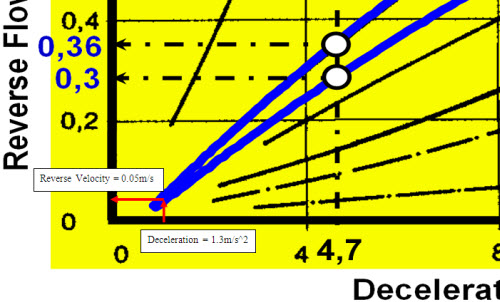Figure 25

Pressure head variation from Surge2000 model – pumps modeled with check valves (linear closure time of 1 s)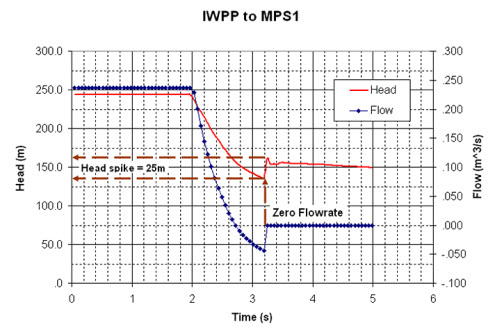Figure 25a

Change in velocity plot for current flow conditions for current flow conditions.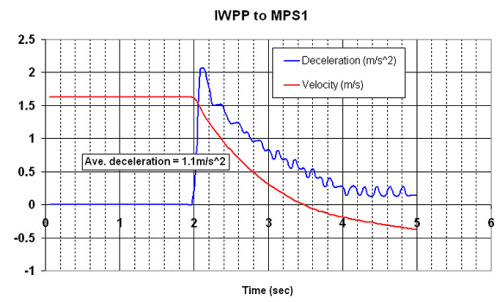Figure 26

Generic closure characteristics of VAG Slanted Seat Swing Check Valves (Courtesy: VAG)